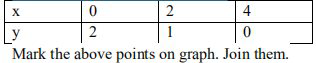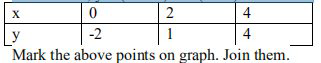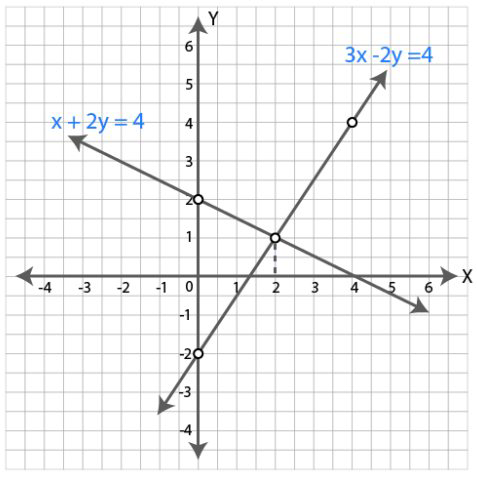# ML Aggarwal Solutions Class 9 Mathematics Solutions for Coordinate Geometry Exercise 19.3 in Chapter 19 - Coordinate Geometry

. Solve graphically the following equations: x + 2y = 4, 3x – 2y = 4

Take 2 cm = 1 unit on each axis. Write down the area of the triangle formed by the lines and the x-axis.

x+2y = 4 …(i)

2y = 4-x

y = (4-x)/2

When x = 0, y = (4-0)/2 = 4/2 = 2

When x = 2, y = (4-2)/2 = 2/2 = 1

When x = 4, y = (4-4)/2 = 0/2 = 03x-2y = 4 ..(ii)

2y = 3x-4

y = (3x-4)/2

When x = 0, y = (3×0-4)/2 = (0-4)/2 = -4/2 = -2

When x = 2, y = (3×2-4)/2 = (6-4)/2 = 2/2 = 1

When x = 4, y = (3×4-4)/2 = (12-4)/2 = 8/2 = 4It is clear from the graph that the two lines intersect at (2,1).

So the solution of the given equations is x = 2 and y = 1.

The area of the triangle formed by these lines and X-axis = ½ ×base×height

= ½ ×2.7×1

= 1.35 sq. units

Hence the area of the triangle is 1.35 sq. units

Related Questions

Lido

Courses

Teachers

Book a Demo with us

Syllabus

Maths
CBSE
Maths
ICSE
Science
CBSE

Science
ICSE
English
CBSE
English
ICSE
Coding

Terms & Policies

Selina Question Bank

Maths
Physics
Biology

Allied Question Bank

Chemistry
Connect with us on social media!